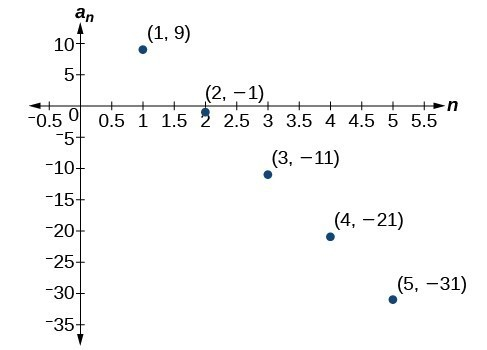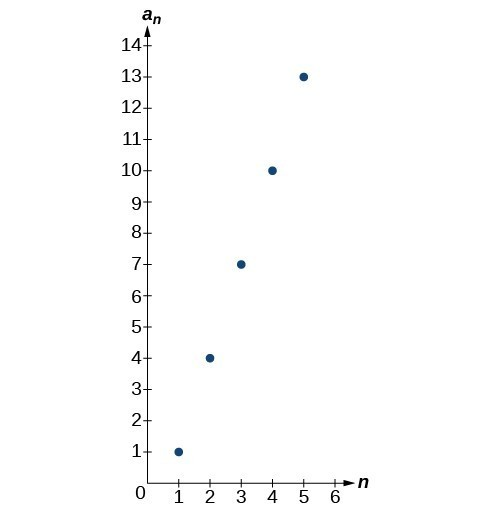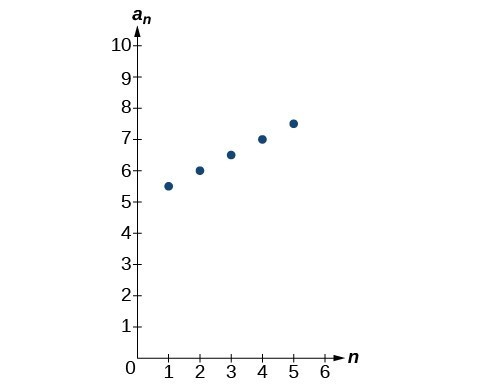## Solutions to Try Its

1. The sequence is arithmetic. The common difference is $-2$.

2. The sequence is not arithmetic because $3 - 1\ne 6 - 3$.

3. $\left\{1, 6, 11, 16, 21\right\}$

4. ${a}_{2}=2$

5. $\begin{array}{l}{a}_{1}=25\hfill \\ {a}_{n}={a}_{n - 1}+12,\text{ for }n\ge 2\hfill \end{array}$

6. ${a}_{n}=53 - 3n$

7. There are 11 terms in the sequence.

8. The formula is ${T}_{n}=10+4n$, and it will take her 42 minutes.

## Solutions to Odd-Numbered Exercises

1. A sequence where each successive term of the sequence increases (or decreases) by a constant value.

3. We find whether the difference between all consecutive terms is the same. This is the same as saying that the sequence has a common difference.

5. Both arithmetic sequences and linear functions have a constant rate of change. They are different because their domains are not the same; linear functions are defined for all real numbers, and arithmetic sequences are defined for natural numbers or a subset of the natural numbers.

7. The common difference is $\frac{1}{2}$

9. The sequence is not arithmetic because $16 - 4\ne 64 - 16$.

11. $0,\frac{2}{3},\frac{4}{3},2,\frac{8}{3}$

13. $0,-5,-10,-15,-20$

15. ${a}_{4}=19$

17. ${a}_{6}=41$

19. ${a}_{1}=2$

21. ${a}_{1}=5$

23. ${a}_{1}=6$

25. ${a}_{21}=-13.5$

27. $-19,-20.4,-21.8,-23.2,-24.6$

29. $\begin{array}{ll}{a}_{1}=17; {a}_{n}={a}_{n - 1}+9\hfill & n\ge 2\hfill \end{array}$

31. $\begin{array}{ll}{a}_{1}=12; {a}_{n}={a}_{n - 1}+5\hfill & n\ge 2\hfill \end{array}$

33. $\begin{array}{ll}{a}_{1}=8.9; {a}_{n}={a}_{n - 1}+1.4\hfill & n\ge 2\hfill \end{array}$

35. $\begin{array}{ll}{a}_{1}=\frac{1}{5}; {a}_{n}={a}_{n - 1}+\frac{1}{4}\hfill & n\ge 2\hfill \end{array}$

37. $\begin{array}{ll}{}_{1}=\frac{1}{6}; {a}_{n}={a}_{n - 1}-\frac{13}{12}\hfill & n\ge 2\hfill \end{array}$

39. ${a}_{1}=4;\text{ }{a}_{n}={a}_{n - 1}+7;\text{ }{a}_{14}=95$

41. First five terms: $20,16,12,8,4$.

43. ${a}_{n}=1+2n$

45. ${a}_{n}=-105+100n$

47. ${a}_{n}=1.8n$

49. ${a}_{n}=13.1+2.7n$

51. ${a}_{n}=\frac{1}{3}n-\frac{1}{3}$

53. There are 10 terms in the sequence.

55. There are 6 terms in the sequence.

57. The graph does not represent an arithmetic sequence.

59.61. $1,4,7,10,13,16,19$

63.65.67. Answers will vary. Examples: ${a}_{n}=20.6n$ and ${a}_{n}=2+20.4\mathrm{n.}$

69. ${a}_{11}=-17a+38b$

71. The sequence begins to have negative values at the 13th term, ${a}_{13}=-\frac{1}{3}$

73. Answers will vary. Check to see that the sequence is arithmetic. Example: Recursive formula: ${a}_{1}=3,{a}_{n}={a}_{n - 1}-3$. First 4 terms: $\begin{array}{ll}3,0,-3,-6\hfill & {a}_{31}=-87\hfill \end{array}$